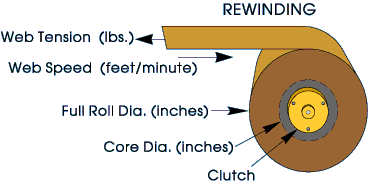## Determining Proper Clutch Size

The proper sized clutch must...

• Have sufficient torque
• Be able to dissipate the heat
• No run above rated speed

### Torque

1) For load simulation, torque limiting and similar applications, torque is already known.

2) For web handling, torque must be calculated. First determine the desired tension in your web (wire, fabric, film etc.).

Calculate Torque: Torque (lb-inches) = Tension (lbs) x Roll Diameter (inches) x 0.5

Use the Full Roll Diameter for calculating the maximum torque needed.  For applications with the web running over a pulley or between nip rollers (pinch rollers), use the pulley diameter as the roll diameter in the formula above.  Always be conservative - select the next larger model if the application requires nearly the rated torque

### RPM (must be less than the maximum allowable)

1) For load simulation, torque limiting and similar applications, RPM is already known.

2) For web handling, usually linear speed (Web Speed) is known, and RPM must be calculated.

Calculate RPM:  RPM = 3.8 x WEB SPEED (feet per minute) / ROLL DIAMETER (inches)

Use the Full Roll Diameter to determine the slowest speed. Use the Core Diameter (empty spool diameter), to determine the fastest speed. For applications with the web running over a pulley or between nip rollers (pinch rollers), use the pulley diameter as the roll diameter.

### Slip Heat Dissipation(model must be physically large enough not to overheat)

1) For any application.

Calculate Heat Input:  HEAT (watts) = TORQUE (lb-inches) x RPM x 0.012

2) For unwinding applications.

Calculate Heat Input:  HEAT (watts) = WEB TENSION (lbs) x WEB SPEED (feet/minute) / 44

To minimize heat input in clutch applications, clutch input RPM should only be about 30-40 RPM faster than the fastest required output RPM. Faster slip speeds generate higher heat and wear with no benefit. Select a gearmotor ratio to achieve this.

For applications with a large percentage change in roll diameter, use an automatic system to control the gearmotor, so the clutch input shaft is always 30 RPM faster than the output shaft. Mount a sensor on each clutch shaft to measure RPM. Connect to the controller. Program to maintain 30 RPM slip. The controller's 0-10v or 4-20mA output can control a variable speed motor drive. Select a varible speed drive to suit your motor.

### Duty Cycle

The average heat input must be below the brake's heat dissipation rating. If the motion is intermittent, use the average speed for thermal (SLIP) calculations.